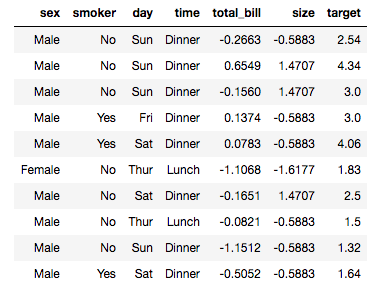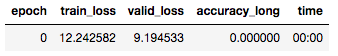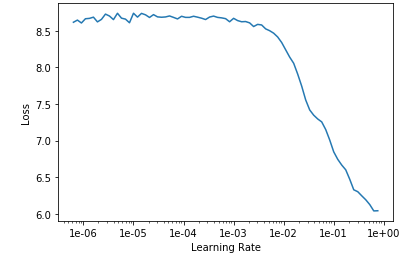# Lesson 4 Tabular dataset with 0% accuracy

Hi, I’m working on the tabular notebook for Lesson 4, using the “A Waiter’s Tips” dataset from Kaggle to predict the tip a waiter will receive based on factors such as the gender of a patron, time of day, day of the week, party size, etc. , and my accuracy rate is exactly 0.

I did have to make my own `accuracy` function, as the default one threw the following error:

Following the advice on this fast.ai forum thread, I defined a new function `accuracy_long` as follows (identical to the default, but with `.long()` appended to the `targs` assignment:

``````def accuracy_long(input:Tensor, targs:Tensor)->Rank0Tensor:
n = targs.shape
input = input.argmax(dim=-1).view(n,-1)
targs = targs.view(n,-1).long()
return (input==targs).float().mean()`
``````

Since the issue is with the accuracy and I had to define my own accuracy function, that seems a likely culprit, but I have no idea how to debug it.

Here’s the notebook, and for further diagnostics, here’s a look at a batch of data:Result of `learn.fit(1, 1e-2)`:I tried running `learn.lr.find()` and plotting:I would sincerely appreciate any tips!

1 Like

It looks to be regression? Perhaps you want to use RMSE like with Rossmann? (Lesson 6)2 Likes

Thanks for the response! I’ve only gotten as far as Lesson 4, so I’ll have to check out Lesson 6.I have my own version of the lesson notebook here and the one for the course is here please let me know if you have questions!1 Like

Thanks again!

1 Like